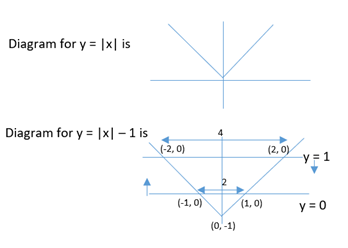# The area of the region satisfying the inequalities | CAT Inequalities

## CAT 2020 - Slot 1 - Quantitative Aptitude - Inequalities - Question 16 - The area of the region satisfying the inequalities

Q.16: The area of the region satisfying the inequalities |x| – y ≤ 1, y ≥ 0, and y ≤ 1 is

|x| – y = 1

y = |x| – 1We got a trapezium with h = 1
And parallel sides = 2 and 4
= 1/2 (2+4) × 1
= 3 square units

### Past Year Question Paper & SolutionsCounselling Session
By IIM Mentor

#### Free Material Area